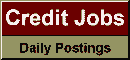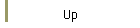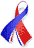DefaultRisk.com the web's biggest credit risk modeling resource.Export citation to:- HTML- Text (plain)- BibTeX- RIS- ReDIF

Bounds for the Distribution of a Multivariate Sum

by Haijun Li of Washington State University,
Marco Scarsini of the Università d'Annunzio, and
Moshe Shaked of the University of Arizona

1996

Abstract:Makarov (1981) and Frank, Nelsen and Schweizer (1987), and independently Rϋschendorf (1982), have found upper and lower bounds for P{X+Y < t} (t Î Â = (-¥, ¥)), when the marginal distributions of X and Y are fixed, and they have proved that their bounds are sharp.

In this paper we find similar bounds when X and Y are vectors rather than scalars. First we determine lower and upper bounds by generalizing the method of Frank, Nelsen and Schweizer and we show that the method can be used also to determine bounds for distributions of functions other than the sum. Then, by generalizing Rϋschendorf's method, based on a theorem of Strassen, we prove that the bounds previously obtained are sharp. Finally we use the bounds to obtain inequalities for expectations of increasing and of Δ-monotone functions of X + Y.

Keywords: Given marginals, Δ-monotone functions, capacities.

Books Referenced in this paper:  (what is this?)